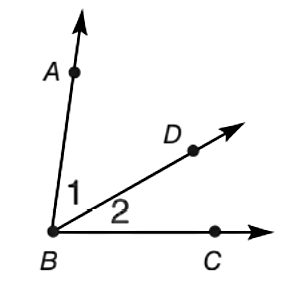Chapter 1.2, Problem 42E### Elementary Geometry for College St...

6th Edition
Daniel C. Alexander + 1 other
ISBN: 9781285195698

#### Solutions

Chapter
Section### Elementary Geometry for College St...

6th Edition
Daniel C. Alexander + 1 other
ISBN: 9781285195698
Textbook Problem
3 views

# In Exercises 41 to 44, m ∠ 1 + m ∠ 2 = m ∠ A B C .Find m ∠ 1 if m ∠ A B C = 68 ° and m ∠ 1 = m ∠ 2 .To determine

To find:

m1 for mABC=68° and m1=m2.

Explanation

Calculation:

Given,

mABC=68° and

m1=m2

m1+m2=mABC

Substitute; mABC=68° and m1=m2 in

### Still sussing out bartleby?

Check out a sample textbook solution.

See a sample solution

#### The Solution to Your Study Problems

Bartleby provides explanations to thousands of textbook problems written by our experts, many with advanced degrees!

Get Started

#### In problems 9-18, simplify the expressions with all exponents positive. 13.

Mathematical Applications for the Management, Life, and Social Sciences

#### Evaluate the expression sin Exercises 116. 3(2)0

Finite Mathematics and Applied Calculus (MindTap Course List)

#### True or False: converges absolutely.

Study Guide for Stewart's Multivariable Calculus, 8th

#### limx0sin5x3x=. a) 1 b) 53 c) 35 d) 0

Study Guide for Stewart's Single Variable Calculus: Early Transcendentals, 8th